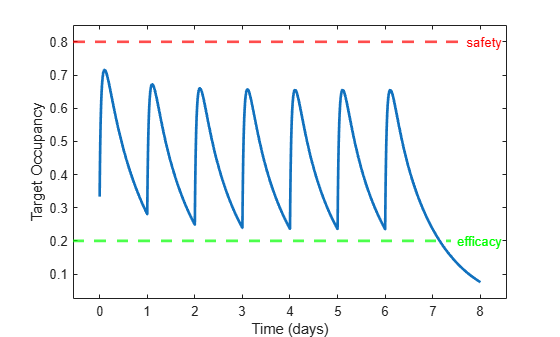# Deploy a SimBiology Model Using SimFunction

This example shows how to compile a SimBiology model using `SimFunction` and MATLAB Compiler and create a web app archive to deploy in MATLAB Web App Server. The example uses a tumor growth model that focuses on the target or receptor occupancy (TO) as a biomarker and explores the dosing efficacy and toxicity.

### Target-Mediated Drug Disposition (TMDD) Model

Target-mediated drug disposition is a phenomenon in which a drug binds with high affinity to its pharmacologic target site, such as a receptor or enzyme, in an interaction that is reflected in the pharmacokinetic characteristics of the drug. For details about the model, see Scan Dosing Regimens Using SimBiology Model Analyzer App.

`sbioloadproject tmdd_with_TO.sbproj;`

### Get Dosing Information

Get the dosing information. The dose object name is Adaptive amount. The object has its amount property parameterized so that you can change the dose amount property by changing another model parameter (Amount).

`dose = getdose(m1,"Adaptive amount")`
```dose = SimBiology Dose Array Index: Name: Type: 1 Adaptive amount repeat ```

Generate a dosing table from the dose object to reuse it later.

`doseTable = getTable(dose)`
```doseTable=1×5 table StartTime Amount Rate Interval RepeatCount _________ __________ ____ ________ ___________ 0 {'Amount'} 0 24 6 ```

### Create SimFunction

Create a `SimFunction` object that creates a function-like interface to let you vary the dose amount and simulate the model response (target occupancy).

```input = "Amount"; output = "TO"; % Suppress informational warning about dosing. warning('off','SimBiology:SimFunction:DOSES_NOT_EMPTY'); simFun = createSimFunction(m1,input,output,dose);```

Accelerate the `SimFunction` object for faster simulation in the deployed app.

```accelerate(simFun); % Restore the warning. warning('on','SimBiology:SimFunction:DOSES_NOT_EMPTY');```

### Simulate Model

Run the `SimFunction` object and check the target occupancy for a given dose amount.

```doseAmount = 100; stopTime = 8; % Simulate for 8 days results = simFun(doseAmount,stopTime,doseTable);```

Plot the target occupancy. Define the safety threshold as 0.8 and efficacy threshold as 0.2.

```lh = plot(results.Time, results.Data, Linewidth=2); yline(0.8,'r--',"safety",LabelVerticalAlignment="middle",Linewidth=2); yline(0.2,'g--',"efficacy",LabelVerticalAlignment="middle",Linewidth=2); ylim([-0.05,1.05]); axis padded xlabel("Time (days)") ylabel("Target Occupancy")```Save `SimFunction` and dosing information as a MAT file to reuse in the deployed app.

`save tmdd_simfun_dosetable.mat simFun doseTable`

### Compile Application and Create Web App Archive

A prebuilt MATLAB app, `app_tmdd_with_TO.mlapp`, has a simple slider to control the dose amount. The app simulates the model each time you change the slider value and plots the corresponding time course of the target occupancy. On startup, the app loads the necessary `SimFunction` and dose table from the MAT file you saved previously. To learn how to build such MATLAB apps, see Develop Apps Using App Designer.

The next steps show you how to compile such an app with additional dependent files needed to create a web app archive that can run on MATLAB Web App Server.

`appfilename = "app_tmdd_with_TO.mlapp";`

To check the dependent files required by `SimFunction`, uncomment the following.

`% simFun.DependentFiles'`

Specify the MAT file and `SimFunction` dependent files as the required dependencies for the app.

`appDependencies = ["tmdd_simfun_dosetable.mat";simFun.DependentFiles'];`

Build the web app archive.

`compiler.build.webAppArchive(appfilename,AdditionalFiles=appDependencies);`

The build function generates a folder named app_tmdd_with_TOwebAppArchive with the necessary files needed for deployment in MATLAB Web App Server. For details, see Deploy Web App (MATLAB Compiler).